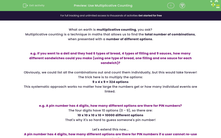# Use Multiplicative Counting

In this worksheet, students will use the method of multiplicative counting to calculate the total number of possible outcomes of linked events, taking into account dependent events (when the result in an earlier choice affects the later choices) or repeated outcomes.Key stage:  KS 4

Year:  GCSE

GCSE Subjects:   Maths

GCSE Boards:   Pearson Edexcel, OCR, Eduqas, AQA,

Curriculum topic:   Number, Probability

Curriculum subtopic:   Structure and Calculation Combined Events and Probability Diagrams

Difficulty level:#### Worksheet Overview

What on earth is multiplicative counting, you ask?

Multiplicative counting is a technique in maths that allows us to find the total number of combinations, when presented with a number of different options.

e.g. If you went to a deli and they had 6 types of bread, 4 types of filling and 9 sauces, how many different sandwiches could you make (using one type of bread, one filling and one sauce for each sandwich)?

Obviously, we could list all the combinations out and count them individually, but this would take forever!

The trick here is to multiply the options:

9 x 4 x 9 = 324 options

This systematic approach works no matter how large the numbers get or how many individual events are linked.

e.g. A pin number has 4 digits, how many different options are there for PIN numbers?

The four digits have 10 options (0 - 9), so there are:

10 x 10 x 10 x 10 = 10000 different options

That's why it's so hard to guess someone's pin number!

Let's extend this now...

A pin number has 4 digits, how many different options are there for PIN numbers if a user cannot re-use a number?

The first digit can be chosen from 10 options.

The second digit can be chosen from 9 options.

The third can be chosen from 8 options.

Finally, the fourth can be chosen from only 7 options.

So all together in this circumstance, a user has:

10 x 9 x 8 x 7 = 5040 options

e.g. Mahlia put the five vowels from the alphabet into a bag and pulls out two. How many different combinations could she make?

If you follow our work from the previous questions, you would think there should be 20 options (5 x 4).

But there's actually only 10!

This is sometimes the case and occurs when the order in which events occur does not matter.

In this question, Mahlia pulls out two vowels so, for example, AE is the same outcome as EA and OU is the same as UO.

When we get this situation, we must to divide our answer by 2, as half of the outcomes are repeated.

In this activity, you will use the method of multiplicative counting described above to calculate the total number of possible outcomes of linked events, taking into account dependent events (when the result in an earlier choice affects the later choices) or repeated outcomes.

### What is EdPlace?

We're your National Curriculum aligned online education content provider helping each child succeed in English, maths and science from year 1 to GCSE. With an EdPlace account you’ll be able to track and measure progress, helping each child achieve their best. We build confidence and attainment by personalising each child’s learning at a level that suits them.

Get started